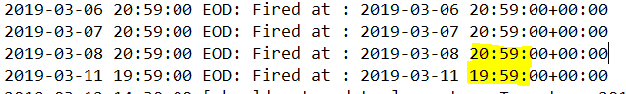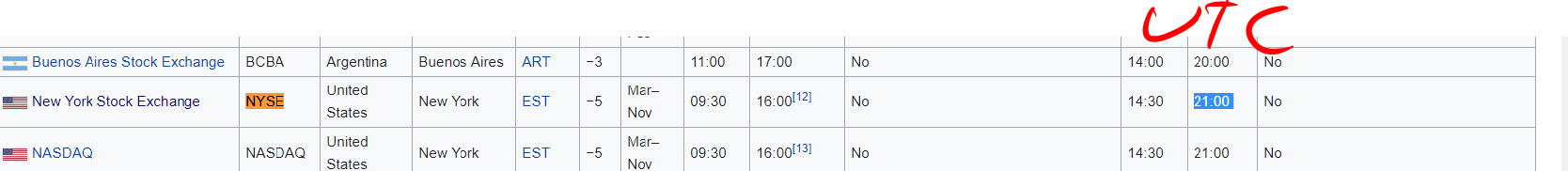Hey all,

I try to create an intraday trading strategy, thus, every EOD I would like to Liquidate my portfolio,

Using Schedule events :

`self.Schedule.On(self.DateRules.EveryDay(), self.TimeRules.BeforeMarketClose("SPY", 1) , Action(self.EOD))def EOD(self):self.Log("EOD: Fired at : {0}".format(self.UtcTime))self.Liquidate()`

and in the alpha itself, I would like to perform a logic only at the first minute of a trading day :

`START_TIME = datetime.time(14, 30, 0)END_TIME = datetime.time(14, 31, 0)# AT THE ALPHA :def Update(self, algorithm, data):if not self.should_get_updates(algorithm):return []#Rest of the logic here `

`return insightsdef should_get_updates(self,algorithm):if time_in_range(START_TIME,END_TIME, datetime.time(algorithm.UtcTime.hour,algorithm.UtcTime.minute, 0) ):algorithm.Debug(f'[should_get_updates] - return True to : {algorithm.UtcTime }')return Trueelse:return Falsedef time_in_range(start, end, x):"""Return true if x is in the range [start, end]"""if start <= end:return start <= x < endelse:return start <= x or x < end`

And actually, it works fine except periods in the year when the EOD and SOD are changed:When checking Wikipedia:Does the UTC time suppose to be changed during the year?

is there any way to emit insights using AlphaModel,Update with external interrupt logic?

I tried to call AlphaModel.Update but seems like the portfolio construction didn't like that and wasn't affected at all

THANKS for the helpers